Welcome to Study Material Solution
Leave Comment

# IMPORTANCE OF MEASUREMENT

In daily life, the importance of measurement is well known. When we have to bring fruits, vegetables etc. from the market then the seller measures their quantity using beam balance and we have to pay him accordingly. The tailor needs exacts measurement of our body to stitch our dresses. We always have an approximation that how much time will be spent on a journey for our convenience. Thus we can conclude that measurement is an essential part of everyday life.
Measurement: It is the determination of the dimensions, quantity or capacity of an item.
Physical quantity: Any quantity that we study in physics (e.g., length, mass and time) is called physical quantity, To measure physical quantities such as mass, time and length, we use units such as kilogram, second, and metre.
Unit: A physical quantity (Such as length) has to be measured with respect to some known quantity. This known quantity is called a unit.

## HISTORY OF LENGTH MEASUREMENT

In ancient times, different body parts were used to measure length. The distance between the tip of the thumb and the tip of the little finger of a fully stretched hand was termed a hand span . The distance between the tip of the middle finger and the elbow was termed a cubit . Foot, stride, fathom , and yard are some more units based on the length of body parts. However, it was soon realized that these units were not very reliable, as the length of body parts can vary from person to person. We need standard units of length and other physical quantities to obtain the same value for a measurement. However, people across the world might adopt different standard units. For example, gram and pound are units of mass used by people of different countries. To solve this problem, we need a set of standard units which is acceptable throughout the world.

### STANDARD SYSTEM OF MEASUREMENT

Standard units are those that have a fixed quantity and, therefore, do not vary from person to person and place to place. For example, the metric system, created by the French in 1790, is a standard set of units. Adopting standard units of measurement solves only half the problem. People in different countries may be using different sets of standard units for measurement. For example. gram and pound set of units. The adoption of SI units has made it easier for scientists of different countries to communicate their results to one another.
The SI system of units was adopted in 1960 by the General conference of Weights and Measures. SI is the short form of system of international Units (in French). The SI unit of length is the metre. Other common standard units of length are inch, millimetre, centimetre, kilometre, and so on.
SI unit of mass and time are kilogram and second respectively. Depending on the size of the object we wish to measure, we have to choose an appropriate unit. For example, we use metres to measure the length of a piece of cloth, kilometres to measure the thickness of the hair, and so on, Units of length can be interconverted by multiplying or dividing their values by 10, 100, 1000, and so on.
A commonly used unit for measuring large distances is kilometer (represented as km.) Commonly used (cm) and millimetre (mm).
1000 m = 1 km
100 cm = 1 m 1000 mm = 1 m
For measuring lengths smaller than the metre, its submultiples are used. For lengths greater than the metre, its multiples are used. Those are given in following table : -Relation between some units:
10 mm = 1 cm, 10 cm = 1 dm, 10 dm = 1m;
10m = 1 dam, 10 dam = 1 hm, 10 hm = 1 km

### MEASUREMENT OF LENGTH

The length of an object is the distance between its ends. It can be measured using a ruler, a measuring tape, a metre rod, and so on whichever is convenient for the required purpose.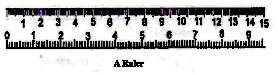### DISTANCE

Measuring the Length of a Curved Line:
We cannot measure the length of a curved line directly by using a metre scale. We can use a thread to measure the length of a curved line.

### Activity

Use a thread to measure the length of the curved line AB. Put a knot on the thread near one of its ends. Place this knot on the point A. Now, place a small portion of the thread along the line, keeping it taut using your fingers and thumb. Hold the thread at this end point with one hand. Using the other hand, stretch a little more portion of the thread along the curved line. Go on repeating this process till the other end B of the curved line is reached. Make a mark on the thread where it touches the end B. Now stretch the thread along a metre scale. Measure the length between the knot in the beginning and the final mark on the thread. This gives the length of the curved line AB.
We see that we need a lot of care to ensure that we are measuring distances and lengths correctly. And, we need some standard units and devices with which we measure these distances and can convey our results to others.
Is the actual path travelled by a body in a given time. Consider a body travelling from A to B along any path between A and B. The actual length of the path that a body travels between A and B is known as the distance. Here if the body goes from A to B via C, the distance travel will be ACB.
The distance travelled will be different for different paths between A and B.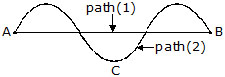### TYPES OF MOTION

You may have observed the motion of a vehicle on a straight road, march-past of soldiers in a parade or the falling of a stone (figure). What kind of motion is this? Sprinters in a 100-metre race also move along a straight track. Can you think of more such examples from your surroundings?
In all these examples we see that the objects move along a straight line. This type of motion is called rectilinear motion.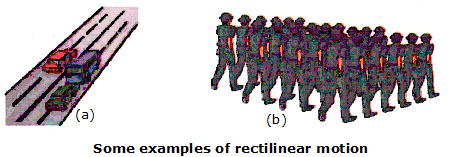### Activity

Take a stone, tie a thread to it and whirl it with your hand. Observe the motion of the stone. We see that the stone moves along a circular path. In this motion, the distance of the stone from your hand remains the same. This type of motion is called circular motion (figure).
The motion of a point marked on the blade of an electric fan or the hands of a clock are examples of circular motion.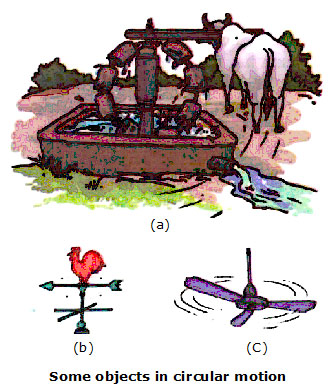Various Graphs Related to Motion :

A. Displacement-Time Graph :
(A) For a stationary body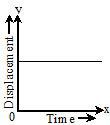(B) For a body moving with constant velocity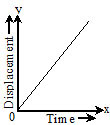(C) For a body moving with non-uniform velocity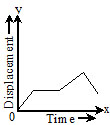(D) For a body with accelerated motion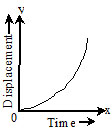(5) For a body with decelerated motion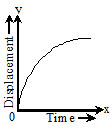(6) For a body which returns towards the point of reference(7) For a body whose velocity constantly changes(8) For a body whose velocity changes after certain interval of timeB. Velocity-Time Graph:
(A) For the body having constant velocity or zero acceleration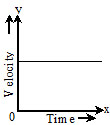(B) When the body is moving with constant retardation and its initial velocity is not zero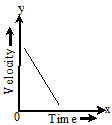(C) When body moves with non-uniform acceleration and its initial velocity is zero.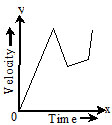(D) When the body is accelerated and its initial
velocity is zero(5) When the body is decelerated.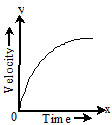C. Acceleration-Time Graph :
(A) When acceleration is constant(B) When acceleration is increasing and is positive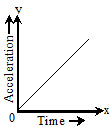(C) When acceleration is decreasing and is negative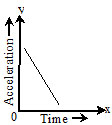(d) When initial acceleration is zero and rate of change of acceleration is non-uniform### OBJECTIVE QUESTIONS

1. The SI unit of length is :
(A) kelvin         (B) metre
(C) ohm            (D) centimetre

2. Speedometer measures
(A) speed
(B) average speed
(C) instantaneous speed
(D) instantaneous velocity

3. The area of rectangle is given as the product of length:
(C) volume             (D) none of these

4. 1 dm is equal to :
(A) 1/10 m             (B) 1/100 m
(C) 100 m              (D) none of these

5. 1 cm3 = ________ m3
(A) 10–6                (B) 10–3               (C) 109               (D) 106

6. What is the correct ascending order ?
(A) 1cm, 1dam, 1dm, 1m
(B) 1cm, 1dam, 1m, 1dm
(C) 1m, 1dm, 1cm,1dam
(D) 1cm, 1dm, 1m, 1dam

7. Motion of the pendulum is :
(A) Linear                          (B) Circular
(C) Rotational                    (D) Oscillatory

8. Motion of the stone dropped under gravity :
(A) Linear                          (B) Circular
(C) Rotational                    (D) Vibrational

9. Motion of the point on blade of a moving fan :
(A) Linear                        (B) Circular
(C) Oscillatory                 (D) Vibrational

10. Motion which repeats itself after a regular
interval of time :
(A) Periodic                     (B) Non Periodic
(C) Rotational                  (D) Vibrational

### SUBJECTIVE QUESTIONS

1. Why handspan and cubit or footstep can't used as standard unit?

2. Distinguish between the state of rest and motion.

3. What are the types of motion?

4. Discuss the used of divider.

5. State the importance of measurements.

1. B            2. C              3. A            4. A           5. A               6. D              7. D                 8. A            9. B              10.A

1. WHich of the following types of motion is not exhibited by the earth moving around the sun?
(A) Circular             (B) Rotational
(C) Rectilinear         (D) Periodic

2. The girth of a tree can be measured by using a:
(A) Plastic rular       (B) Metre scale
(C) netre rod            (D) Measuring tape

3. WHich of the following modes of transport is not based on the invention of wheel?
(A) Bullock cart      (B) Boat
(C) Bicycle              (D) Bus

4. WHich of the following types of motion are possessed by a merry go round at the same time?
(i) Rotational                    (ii) Rectilinear motion
(iii) Periodic Motion        (iv) Circular Motion
(A) (i) and(ii)                   (B) (ii) and (iii)
(C) (i) and (iii)                 (D) (i) and (iv)

5. A metre scale can measure lengths accuratey up to:
(A) 1 cm                           (B) 1 mm
(C) 1 Km                          (D) 1 m

6. Which of the following types of motion are possessed by a football rolling on the ground?
(i) Rotational Motion
(ii) Rectilinear motion
(iii) Circular Motion
(iv) Periodic Motion
(A) (i) and (ii)                    (B) (ii) and (iii)
(C) (i) and (iii)                   (D) (ii) and (iv)

7. The distance between Anuskha's home and Devika home is 1425 m. This distance is equal to
(A) 14.25 Km                    (B) 142.5 Km
(C) 1.425 Km                    (D) 0.1425 Km

8. A communication satellite us stational in an orbit high above the earth. Which of the fol lowing will not be exhibited by this communi cation satellite?
(i) Rotational Motion              (ii) Periodic Motion
(iii) Circular Motion               (iv) Rectilinear Motion
(A) (i) and (ii)                         (B) (ii) and (iii)
(C) (i) and (iv)                        (D) (ii) and (iv)

9. The Most concenient unit for measuring the thickness of a coin is
(A) Centimetre                        (B) Kilometre
(C) Metre                                 (D) Millimetre
10. A bicycle is moving on a straight road. Which of the following types of motion are exhibited by it?
(i) Periodic motion                  (ii) Rotational Motion
(iii) Rectilinear Motion           (iv) Circular Motion
(A) (i) and (ii)                         (B) (ii) and (iii)
(C) (iii) and (iv)                      (D) (i) and (iv)

11. The height of a man is 1.56 m. This height is equal to
(A) 1560mm                          (B) 156mm
(C) 15.6 mm                          (D) 1560 cm

12. One of the foolowing types of motion will not be exhibited by the moon moving around the earth. This one is
(A) Rotational                       (B) Rectilinear
(C) Circular                          (D) Periodic

13. WHich one of the following is the smallest unit of length?
(A) Km                                 (B) mm
(C) cm                                   (D) m

14. The tip of the seconds hand of a clock is coloured red. WHich of the following types of motion will be exhibited by the red coloured tip of the second hand of this clock
(i) Rotational Motion (ii) Periodic Motion
(iii) Circular Motion (iv) Rectilinear Motion
(A) (i) and (ii)                       (B) (i) and (iii)
(C) (ii) and (iii)                     (D) (iii) and (iv)

1. C          2. D               3. B          4. D       5. B              6. A                   7. C              8. C       9. D           10. B                11. A              12. B
13. B       14. C

## SECTION -A

### Fill in the following blanks with suitable words:

(i) The length of forearm from elbow to finger tips is called...........

(ii) Every measurement consists of a number and a .....................

(iii) THe change is position of an object with time is called.................

(iv) A falling stone exhibits.............motion

(v) One metre is ....................mm

### SECTION-B

1. Explain why, hand-span cannot be used as a standard unit of lenght.

2. WHat is cubit? Explain why cunit cannot be used as a standard unit of lenght

3. WHy a foot step cannot be used as a standard unit of lenght?

4. Write the full names of the following units of measurement
(a) cm                    (b) km                          (c) mm                 (d) m

5. Write the abbreviations for the following units
(a) centimetre        (b) metre                       (c) Kilometre       (d) Millimetre

6. What is meant by rectilinear motion? Given two examples of rectilinear Motion.

7. What is periodic motion? State two examples of periodic or rotational motion.

8. Name the two modes of transport used
(a) on land               (b) in water                   (c) by air

SECTION - A

1. (i) Cubic             (ii) Unit                (iii) Motion                 (iv) rectilinear                (v) 1000